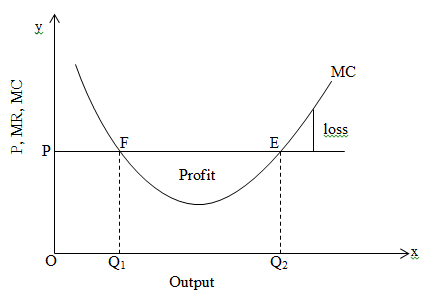# Theory of product pricing

Firm and industry:
Firm is a business unit for a particular commodity and industry ids a group of firms which produces same commodity.

Equilibrium of a firm:
Equilibrium refers to the condition where a firm gets maximum revenue and minimum loss. In this condition, there is no tendency of change of level of output. Firm do not want to increase or decrease the level of output under this condition. In all kinds of market structure, firm gets equilibrium if there are:

a. marginal cost (MC) equal to marginal revenue (MR)
b. marginal cost curve cuts to the marginal revenue curve from below.

Equilibrium of a firm under perfect competition market (MC and MR approach):
Under the perfect competition market MR curve is parallel to x-axis. In this market also, a firm can achieve equilibrium position when given two conditions are fulfilled:

a. when MC = MR
b. MC cuts MR from below

By the help of given figure we can explain equilibrium of a firm under perfect competition market.On the above figure, output is measured on x-axis whereas price, marginal revenue and marginal cost is measured on y-axis. At point F, MR and MC are equal and MC cuts MR from above so that point F is not the equilibrium point because if the producer produces more than Q1 then profit amount is increased. So, he wants to produce more which is against equilibrium position. But point E is equilibrium position because if he produces more than Q2 there is no chance of profit. So, producer wants to remain at point E by producing Q2 output which gives maximum revenue and MC = MR when MC cuts MR from below.